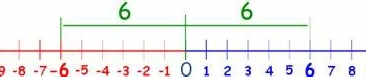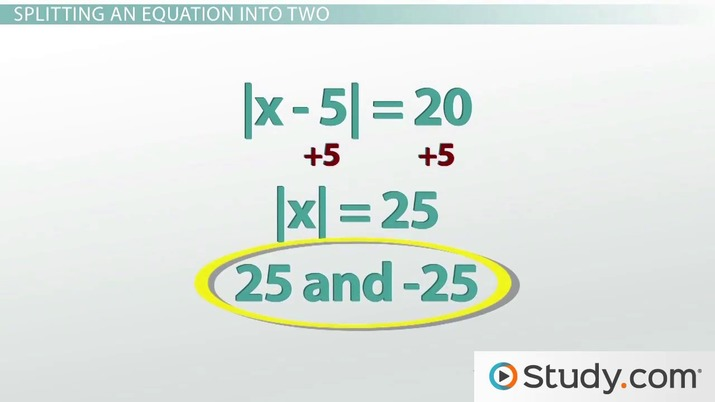# Solving Absolute Value Equations

Shavonne McNary, Zach Pino
• Author
Shavonne McNary

Shavonne McNary has taught and tutored a combination of 8th grade mathematics, secondary, and post-secondary mathematics courses for over 10 years. She holds a Master’s degree in Adult and higher education with a concentration in mathematics instruction, a Bachelor’s degree in Mathematical Sciences with a minor in secondary education, and an Associate’s degree in Engineering. Shavonne also holds a State Board of Education secondary 8-12 mathematics teaching certification.

• Instructor
Zach Pino
Discover how to simplify and solve absolute value expressions for the value of x. Learn the rules of operations and how to make an absolute value equation. Updated: 03/07/2022

Show

## How to Solve Absolute Value Functions

The term absolute value is used to describe the distance between any point on a number line and zero.Absolute value numbers are written in nonnegative terms. For example:

|-5| = 5 and |5| = 5; |-13| = 13 and |13| = 13

The value inside the absolute value symbols can be either positive or negative and will follow the general rule of

{eq}|x|>=0 {/eq}

where x = k or x = -k. Any absolute value equation will result in two solutions, and the content inside the absolute value symbols can include constants, variables, or expressions. Here we will learn how to solve absolute value functions, but first, some rules.An error occurred trying to load this video.

Try refreshing the page, or contact customer support.

Coming up next: Solving Absolute Value Practice Problems

### You're on a roll. Keep up the good work!

Replay
Your next lesson will play in 10 seconds
• 0:10 Review of Absolute Values
• 0:51 Solving Basic…
• 2:21 Splitting an Equation into Two
• 5:02 Lesson Summary
Save Save

Want to watch this again later?

Timeline
Autoplay
Autoplay
Speed Speed

## Rules Before Performing Operations with Absolute Value

Before performing operations with absolute values, remember to perform the operation inside of the absolute value symbols first (if possible). Then perform additional operations according to the order of operations model (PEMDAS/GEMDAS). Absolute value symbols are considered grouping symbols like parentheses, so the terms inside them must be performed first if they are like terms.

Performing operations with absolute value symbols is also referred to as simplifying absolute value expressions, which means to write the expression without the absolute value symbols. Applying the absolute value operation means to change the single value between the absolute value symbols to positive. Then continue to perform any additional operations according to PEMDAS/GEMDAS.

a) |3 - 5| = |-2| = 2

b) -|9| = -9

c) |6| - |-5| = 6 - 5 = 1

## Solving Absolute Value Equations for x

When performing operations with absolute value expressions involving variables, positive ({eq}x >= 0 {/eq}) and negative ({eq}x < 0 {/eq}) outcomes must be taken into consideration. To write |x - 7|, without using the absolute value symbols, we have the following conditions:

• if x - 7 >= 0, then |x - 7| = x - 7
• if x - 7 < 0, then |x - 7| = -(x - 7) = -x + 7 = 7 - x

Meaning that we want the solution going in the positive direction of x - 7 units away from the origin (zero) and the negative direction x - 7 units away from the origin (zero).

## How to Make an Absolute Value Equation

Absolute value equations can be written from solutions. When given solutions in the form x = {-18, 18} or x = +/- 18, this can be written with an absolute value equation |x| = 18.

The following examples provides some practice on how to make an absolute value equation from solutions.

a) x = +/- 12 --> x = 12 and x = -12 --> |x| = 12

b) x = +/- 3 --> x = 3 and x = -3 --> |x| = 3

Absolute value equations can be in the form {eq}|x| = k {/eq} where {eq}x = k {/eq} or {eq}x = -k {/eq}, meaning that the solution to an absolute value equation can either be positive or negative.

Before solving absolute value equations, make sure that the equation is not equal to a value less than 0 on the right side of the equation. If so, there is no solution.

When solving absolute value equations, remember that absolute value equations:

• Cannot have a negative outside of the absolute value symbol, like -|x|.
• Cannot have anything added to or subtracted from the absolute value symbol, like |x| - 2.
• Cannot have a coefficient greater than 1, like 5|x|.

a) {eq}|x| = 15 {/eq}

Step 1: Set up the absolute value equation for a positive and negative solution.

• {eq}|x| = 15 {/eq} and {eq}|x| = -15 {/eq}

Step 2: Drop the absolute value symbols.

• {eq}x = 15 {/eq} and {eq}x = -15 {/eq}

## Solving Absolute Value Equations

In the following absolute value equations, expressions are inside the absolute value symbols.

a) {eq}|x + 11| = 3 {/eq}

Step 1: Set up the absolute value equation for a positive and negative solution.

• {eq}|x + 11| = 3 {/eq} and {eq}|x + 11| = -3 {/eq}

Step 2: Drop the absolute value symbols.

• {eq}x + 11 = 3 {/eq} and {eq}x + 11 = -3 {/eq}

Step 3: Solve both equations for x by performing opposite operations to isolate the variable.

To unlock this lesson you must be a Study.com Member.

#### How do you solve an absolute value function?

Absolute value functions that contain one constant, we apply the rule of absolute value by dropping the parenthesis and write the value as a positive number. Functions that contain one or more terms in the form of constants, ex. |-5 + 3|, We simplify the value inside of the absolute value symbol, drop the absolute value symbol, and write the value as a positive number.

#### What are the 3 major steps to solving an absolute value equation?

To solve absolute value equations, you write the equation with a positive and a negative value of k, drop the absolute value symbols, and solve each equation for x. Check each solution to see if it makes a true absolute value statement.

### Register to view this lesson

Are you a student or a teacher?